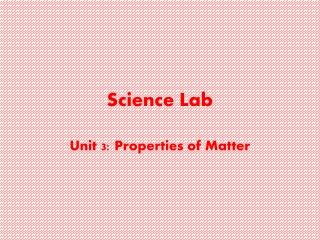Download PresentationScience Lab

# Science Lab

Download Presentation## Science Lab

- - - - - - - - - - - - - - - - - - - - - - - - - - - E N D - - - - - - - - - - - - - - - - - - - - - - - - - - -
##### Presentation Transcript

1. Science Lab Unit 3: Properties of Matter

2. 07-PS1-2 I can identify and classify matter using their physical properties. I can identify and describe the evidence of chemical and physical changes in matter. I can determine if a reaction has occurred by analyzing properties of a substance.

3. Properties of Matter • Mass • Volume • Denstiy • Solubility • Melting & Boiling Point • State of Matter • Metallic Properties • Length • Other: Shape, Size, and Color

4. Mass • Definition • the amount of matter in an object • How do we Measure? • Triple Beam Balance • Digital Scale • Mass of a Liquid • Use a graduated cylinder and take the mass of the empty cylinder • Pour liquid in and then measure the graduate cylinder again • Take the difference= mass of the liquid • Mass of a solid • Simply place object on balance or digital scale. • Unit • Grams • 1000mg=1 gram • 1000g=1 kg ( Mini-Lab Mass of a solid, Mass of Liquid)

5. Volume • Definition • The amount of space an object takes up • How do we Measure? • Ruler, graduated cylinder • Volume of a liquid • Simply read the graduated cylinder • Unit- mL (milliliter) • Volume of a Regular shaped object • Use a ruler and measure the length, width, and height of the object • L x W x H • Unit- cmᶟ • Volume of an irregular sized object • Water Displacement Test • Fill a graduated cylinder up to a desired amount. Usually 100 mL • Place the object into the water and take a reading on graduated cylinder • Subtract 100mL from the reading = volume of an irregular sized object • Unit- cmᶟ (Lab- How to Measure Volume)

6. Density • Definition • the amount of mass a material has in a given volume • D=M/V • How do we measure? • Ruler, graduated cylinder, scale or triple beam balance • Density of a solid and liquid • Take the mass of the object on the scale. • Take the volume of the object. • Refer to Volume notes on how to do so. • Mass divided by volume • Unit- g/cmᶟ or cubic mL • Comparing densities of different liquids • Build a density column (Cover Density PPT activity and Liquid Layer Lab and Density for Solids Lab)

7. Solubility • Definition • The number of grams of one substance will dissolve in 100g of another substance at a given temperature. • How do we measure? • Hot plate, stirrer, beaker, thermometer (Dissolving Rates and Solutions Lab)

8. Melting Point & Boiling Point • Definition • Melting point- the temperature at which a solid changes to liquid • MP of Water= 0°C • Boiling Point- the temperature at which a liquid changes to gas. • BP of water=100°C • It does NOT depend on the size of the sample the MP and BP are ALWAYS the same.